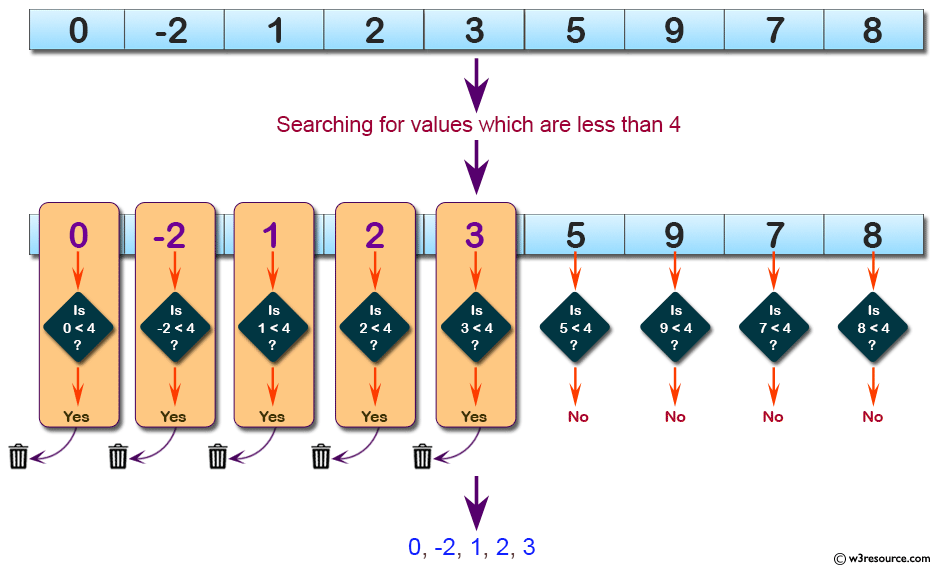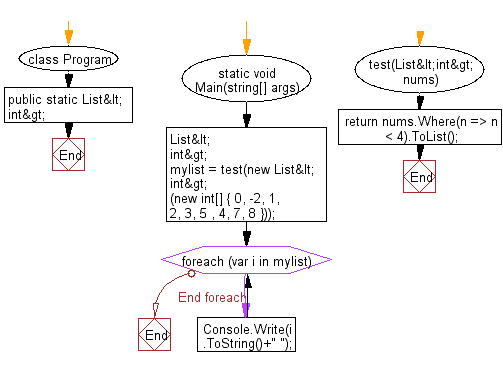﻿ C# - Remove all a in each string in a list of strings# C# Sharp Basic Algorithm Exercises: Create a new list from a given list of integers removing those values which are less than 4

## C# Sharp Basic Algorithm: Exercise-149 with Solution

Write a C# Sharp program to create a new list from a given list of integers removing those values which are less than 4.

Pictorial Presentation:Sample Solution:-

C# Sharp Code:

``````using System;
using System.Collections.Generic;
using System.Linq;
namespace exercises
{
class Program
{
static void Main(string[] args)
{
List<int> mylist = test(new List<int>(new int[] { 0, -2, 1, 2, 3, 5 , 4, 7, 8 }));
foreach(var i in mylist)
{
Console.Write(i.ToString()+" ");
}
}
public static List<int> test(List<int> nums)
{
return nums.Where(n => n < 4).ToList();
}
}
}

```
```

Sample Output:

`0 -2 1 2 3`

Flowchart:C# Sharp Code Editor:

Improve this sample solution and post your code through Disqus

What is the difficulty level of this exercise?

Test your Programming skills with w3resource's quiz.

﻿